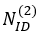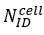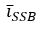5G/NR - PBCH Decoding Process                              Home : www.sharetechnote.com PBCH Decoding Process   When you power on 5G/NR UE(NR Standalone UE) or When LTE is adding NR Cell as a secondary cell, the first thing happening in UE is the process described below. This summary is based on Matlab 5G Toolbox example (Ref ) and the implementation on UE may vary a little bit from this. i) Search Frequency in which SSB (PSS + SCC + PBCH) is transmitted. ii) Search PSS ==> By detecting PSS, UE can figure out. This is done in time domain by calculating correlation between each reference NID(2) sequences with the received signal. With the correlation calculation, we can figure out the exact timing offset(i.e, exact symbol start timing of PSS) in addition to finding out the best NID(2). This timing value (let's call it as 'offset') is the crucial information to construct the accruate resource grid that is used in step iii) and onwards. iii) Construct the resource grid from the received signal and the detected timing from step ii) iv) Search SSS by correlating the extracted SSS REs and calculating the correlation between the extracted SSS and Reference SSS ==> By detecting SSS, UE can figure outv) Fromand, UE can calculate Physical Cell ID ()   v) Search PBCH DMRS ==> from PBCH DMRS UE can estimate channel ==> Calculate the channel matrix H. This will be used for equalization estimate noise figure outvi) (based on Channel and Noise estimation) Perform Equalization(MMSE) for PBCH extraction ==> Once this equalization is done properly, UE can figure out RE for PBCH figure out PBCH CSI vii) Demodulate PBCH ==> figure out PBCH bits   viii) Decode PBCH ==> Figure out MIB       Reference